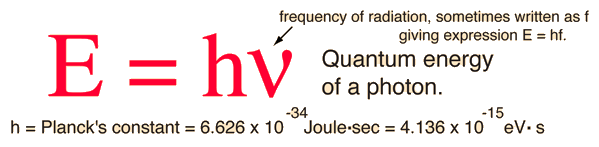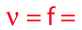# Early Photoelectric Effect Data

Electrons ejected from a sodium metal surface were measured as an electric current. Finding the opposing voltage it took to stop all the electrons gave a measure of the maximum kinetic energy of the electrons in electron volts.The minimum energy required to eject an electron from the surface is called the photoelectric work function. The threshold for this element corresponds to a wavelength of 683 nm. Using this wavelength in the Planck relationship gives a photon energy of 1.82 eV.

### Further analysis

 Table of photoelectric effect work functions
Index

Millikan reference

Photoelectric effect

 HyperPhysics***** Quantum Physics R Nave
Go Back

# Early Photoelectric Effect Data### Planck hypothesis

Index

Millikan reference

Photoelectric effect

 HyperPhysics***** Quantum Physics R Nave
Go Back

# The Planck Hypothesis

In order to explain the frequency distribution of radiation from a hot cavity (blackbody radiation) Planck proposed the ad hoc assumption that the radiant energy could exist only in discrete quanta which were proportional to the frequency. This would imply that higher modes would be less populated and avoid the ultraviolet catastrophe of the Rayleigh-Jeans Law.The quantum idea was soon seized to explain the photoelectric effect, became part of the Bohr theory of discrete atomic spectra, and quickly became part of the foundation of modern quantum theory.

### Calculation

Interaction of radiation with matterElectromagnetic spectrumBasic quantum processes

### Are there limits on the frequency of a photon?

Index

Photoelectric effect

 HyperPhysics***** Quantum Physics R Nave
Go Back

# Photon Energies for EM SpectrumQuantum energy hυ = hf = x 10^ eV Quantum energy hυ = hf = eV =MeV =GeV

### Wavelengthx 10^m

= m
= nm
= microns

### Frequencyx 10^Hz

= kHz = MHz
= GHz
= 1/cm (wavenumber)

### Thermal energy for comparison

Index

 HyperPhysics***** Quantum Physics R Nave
Go Back

# Photons: The Quanta of Light

According to the Planck hypothesis, all electromagnetic radiation is quantized and occurs in finite "bundles" of energy which we call photons. The quantum of energy for a photon is not Planck's constant h itself, but the product of h and the frequency. The quantization implies that a photon of blue light of given frequency or wavelength will always have the same size quantum of energy. For example, a photon of blue light of wavelength 450 nm will always have 2.76 eV of energy. It occurs in quantized chunks of 2.76 eV, and you can't have half a photon of blue light - it always occurs in precisely the same sized energy chunks.

But the frequency available is continuous and has no upper or lower bound, so there is no finite lower limit or upper limit on the possible energy of a photon. On the upper side, there are practical limits because you have limited mechanisms for creating really high energy photons. Low energy photons abound, but when you get below radio frequencies, the photon energies are so tiny compared to room temperature thermal energy that you really never see them as distinct quantized entities - they are swamped in the background. Another way to say it is that in the low frequency limits, things just blend in with the classical treatment of things and a quantum treatment is not necessary.### Calculation

Interaction of radiation with matterElectromagnetic spectrumBasic quantum processes
Index

Photoelectric effect

 HyperPhysics***** Quantum Physics R Nave
Go Back